# How to Apply Conditional Formatting across Workbook in Excel

Sometimes we list all products/students or other objects information in one workbook, but list part of them into another workbook based on some criteria. For example, we list all student’s information into workbook Book1 Sheet-All, and list the students who pass the exam in Book2 Sheet-Pass. If we want to lookup students in Book1 refer to the list in Book2, we can apply conditional formatting function to mark these students with proper format.

Precondition:

Table1 in Book1 Sheet-All.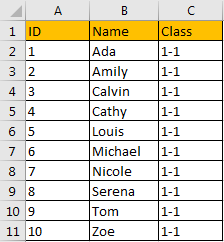Table2 in Book2 Sheet-Pass.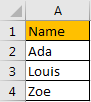Now, we want to find out matched values in table1 based on values in table2.

## Method: Apply Conditional Formatting across Workbook

Step 1: In this step, you can copy table2 from Book2 to Book1 Sheet-All just next to table1. Or you can type the formula ='[Book2.xlsx]Sheet-Pass’!A2 into any cell next to table1 to get values from Book2 table2. Drag fill handle down till 0 displays.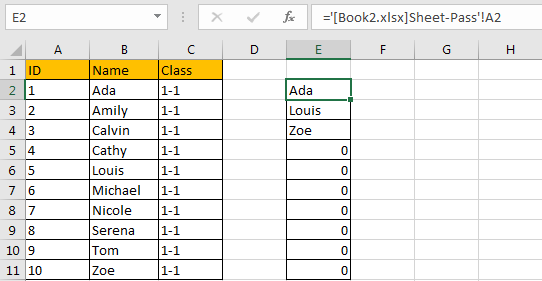Now all names exist in table2 from another workbook/worksheet are copied into current workbook/worksheet.

Step 2: Select table1, this table is the range we want to apply conditional formatting function. Click Home in ribbon, then click Conditional Formatting in Styles group, select New Rule in menu.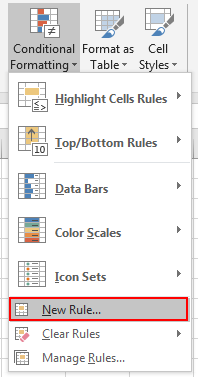Step 3: On New Formatting Rule dialog, select the last rule type: Use a formula to determine which cells to format.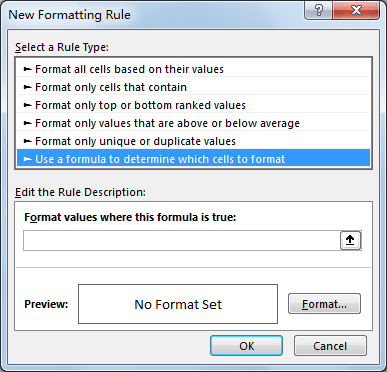Step 4: In Edit the Rule Description section, in Format values where this formula is true textbox, enter this formula =COUNTIF(\$E\$2:\$E\$4,\$B2)>0. In this formula, \$E\$2:\$E\$4 is the range you refer to from table2 (just copied to current worksheet), \$B2 is the first name you want to look up from another workbook.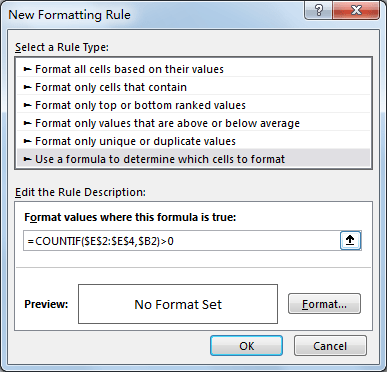Step 5: Click Format button in Preview section. Verify that Format Cells window is displayed.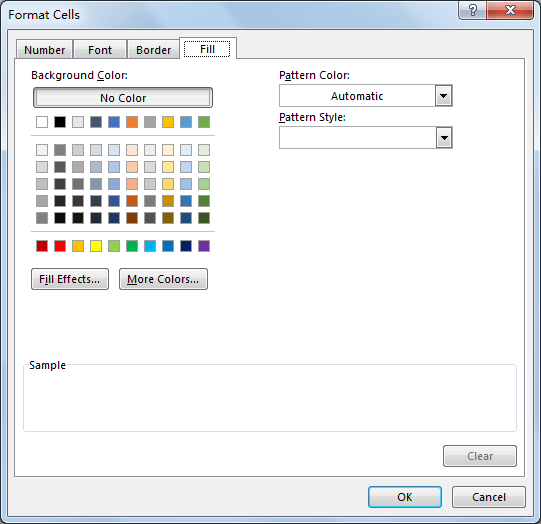Step 6: Click Fill tab, then select a color as background color. For example, select yellow, then click OK.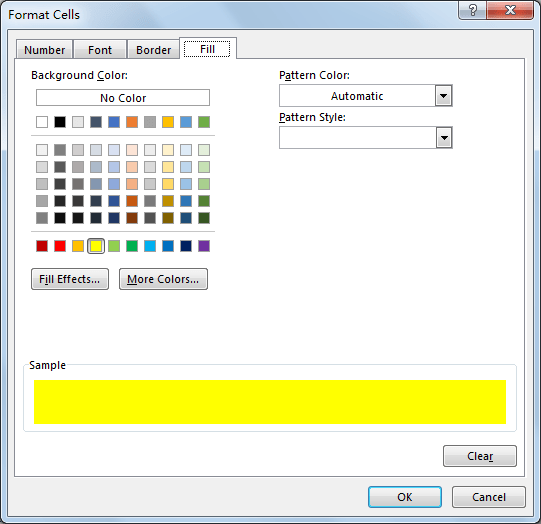Step 7: After above step, we go back to New Formatting Rule window. You can see that in Preview section, cell is filled with yellow. Click OK.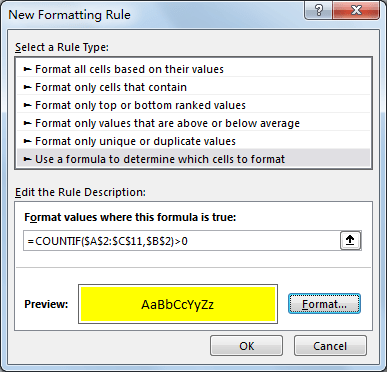Step 8: After above all steps, check table1. Verify that matched values are marked with yellow properly.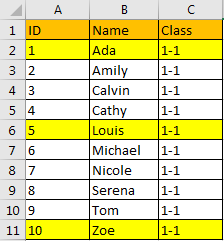Related Posts

If Cell is This Value or That Value

IF function is frequently used in Excel worksheet to return you expect “true value” or “false value” based on the result of logical test. If you want to see if a cell is A or B, and if one of ...

If Value is Greater Than A Certain Value

IF function is frequently used in Excel worksheet to return you expect “true value” or “false value” based on the logical test result. If you want to see if a value in one cell is greater than a specific value, ...

If Cell is Not Blank

IF function is frequently used in Excel worksheet to return you expect “true value” or “false value” based on the result of created logical test. If you want to see if a cell is blank or not, and leave some ...

VBA Macro For VLOOKUP From Another Sheet

In the previous post, you should know that how to fix or remove the #N/A error when using VLOOKUP formula to lookup value from another sheet. And this post will show you how to use VBA code to vlookup data ...

If Cell is Blank

IF function is frequently used in Excel worksheet to return you expect “true value” or “false value” based on the result of created logical test. If you want to see if a cell is blank or not, and leave some ...

If Cell Equals Certain Text String

IF function is frequently used in Excel worksheet to return you expect “true value” or “false value” based on the result of created logical test. If you want to see if cell equals a certain text string like “Win”, you ...

If Cell Contains Either Text1 or Text2

IF function is frequently used in Excel worksheet to return “true value” or “false value” based on the logical test result. If you want to see if cell contains certain substring1 like “abc” or substring2 like “def”, and returns true ...

If Cell Contains Certain Text OR Equals Certain Text

IF cell equals certain text IF function is frequently used in Excel worksheet to return “true value” or “false value” based on the logical test result. If you want to test values to see if they equal certain text like ...

VLOOKUP From Another Sheet Not Working

In the previous post, you should know that how to fix or remove the #N/A error when using VLOOKUP formula to lookup value from another sheet. And this post will show you reasons why your VLOOKUP formula is not working ...

If Cell Begins with One of Three Supplied Characters

If you want to test values to see if they begin with some given specific characters like “x”, ”y”, or “z”, you can create a formula with COUNTIF and SUM functions to return results. EXAMPLE You can see “TRUE” or ...

Sidebar